# MP Board Class 6th Maths Solutions Chapter 12 Ratio and Proportion Ex 12.2

## MP Board Class 6th Maths Solutions Chapter 12 Ratio and Proportion Ex 12.2

Question 1.
Determine if the following are in proportion.
(a) 15, 45, 40, 120
(b) 33, 121, 9, 96
(c) 24, 28, 36, 48
(d) 32, 48, 70, 210
(e) 4, 6, 8, 12
(f) 33, 44, 75, 100
Solution: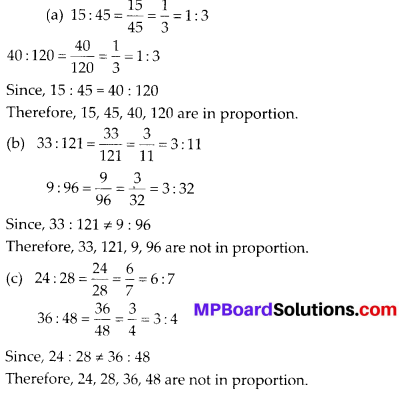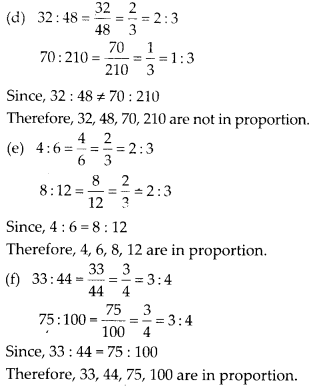Question 2.
Write True (T) or False (F) against each of the following statements:
(a) 16 : 24 :: 20 : 30
(b) 21 : 6 :: 35 : 10
(c) 12 : 18 :: 28 : 12
(d) 8 : 9 :: 24 : 27
(e) 5.2 : 3.9 :: 3 : 4
(f) 0.9 : 0.36 :: 10 : 4
Solution:
(a) True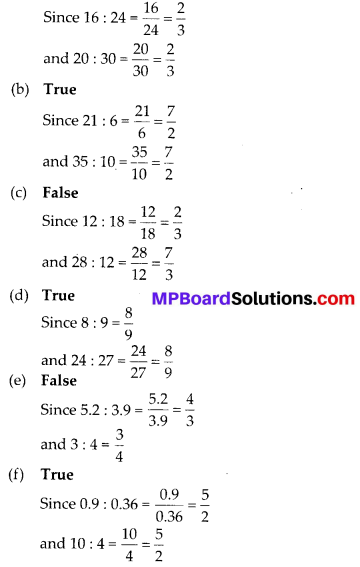Question 3.
Are the following statements true?
(a) 40 persons : 200 persons = Rs. 15 : Rs. 75
(b) 7.5 litres: 15 litres = 5 kg : 10 kg
(c) 99 kg : 45 kg = Rs. 44 : Rs. 20
(d) 32 m: 64 m = 6 sec : 12 sec
(e) 45 km : 60 km = 12 hours : 15 hours
Solution:
(a) 40 persons : 200 persons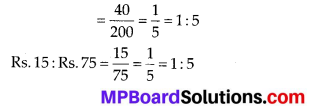∴ 40 persons : 200 persons = Rs. 15 : Rs. 75
Hence, the statement is true.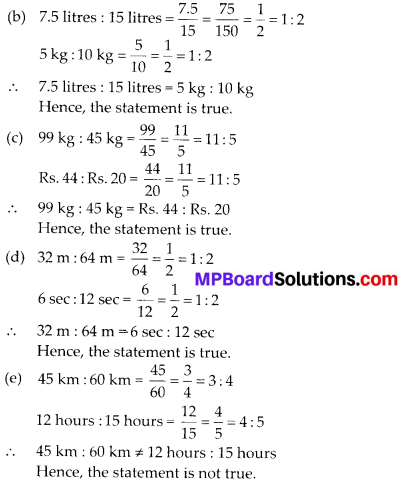Question 4.
Determine if the following ratios form a proportion. Also, write the middle terms and extreme terms where the ratios form a proportion.
(a) 25 cm : 1 m and Rs. 40: Rs. 160
(b) 39 litres: 65 litres and 6 bottles: 10 bottles
(c) 2 kg : 80 kg and 25 g : 625 g
(d) 200 ml: 2.5 litre and Rs. 4: Rs. 50
Solution:
(a) 25 cm : 1 m = 25 cm : (1 × 100) cm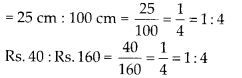Since the ratios are equal, therefore these are in proportion.
Middle terms are 1 m and Rs. 40 and extreme terms are 25 cm and Rs. 160.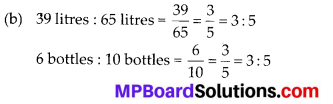Since the ratios are equal, therefore these are in proportion.
Middle terms are 65 litres and 6 bottles and extreme terms are 39 litres and 10 bottles.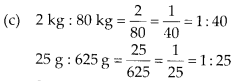Since the ratios are not equal, therefore these are not in proportion.
(d) 200 ml : 2.5 litres = 200 ml: (2.5 × 1000) ml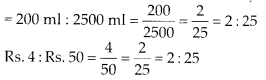Since the ratios are equal, therefore these are in proportion.
Middle terms are 2.5 litres and Rs. 4 and extreme terms are 200 ml and Rs. 50.